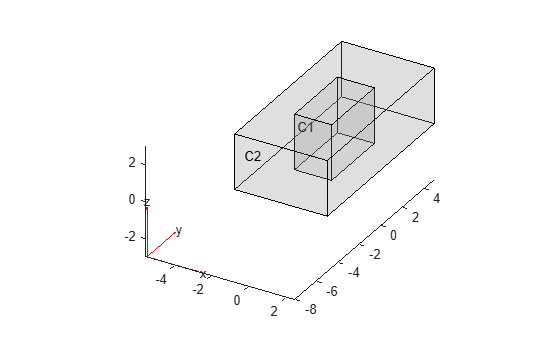# cellFaces

Find faces belonging to specified cells

Since R2021a

## Syntax

``FaceID = cellFaces(g,RegionID)``
``FaceID = cellFaces(g,RegionID,FilterType)``

## Description

example

````FaceID = cellFaces(g,RegionID)` finds faces belonging to the cells with ID numbers listed in `RegionID`.```

example

````FaceID = cellFaces(g,RegionID,FilterType)` returns internal, external, or all faces belonging to the cells with ID numbers listed in `RegionID`.```

## Examples

collapse all

Find faces belonging to two cuboids in a geometry consisting of four stacked cuboids.

Create a geometry that consists of four stacked cuboids.

`gm = multicuboid(5,10,[1 2 3 4],"ZOffset",[0 1 3 6])`
```gm = DiscreteGeometry with properties: NumCells: 4 NumFaces: 21 NumEdges: 36 NumVertices: 20 Vertices: [20x3 double] ```

Plot the geometry with the cell labels.

`pdegplot(gm,"CellLabels","on","FaceAlpha",0.2)`Find faces belonging to cells 1 and 3.

`faceIDs = cellFaces(gm,[1 3])`
```faceIDs = 1×12 1 2 3 4 5 6 7 12 13 14 15 16 ```

Plot the geometry with the face labels.

`pdegplot(gm,"FaceLabels","on","FaceAlpha",0.2)`Find faces belonging to the outer cuboid in a geometry consisting of two nested cuboids.

Create a geometry that consists of two nested cuboids of the same height.

`gm = multicuboid([2 5],[4 10],3)`
```gm = DiscreteGeometry with properties: NumCells: 2 NumFaces: 12 NumEdges: 24 NumVertices: 16 Vertices: [16x3 double] ```

Plot the geometry with the cell labels.

`pdegplot(gm,"CellLabels","on","FaceAlpha",0.2)`Find all faces belonging to the outer cell.

`faceIDs = cellFaces(gm,2)`
```faceIDs = 1×10 3 4 5 6 7 8 9 10 11 12 ```

Find only the internal faces belonging to the outer cell. Internal faces are faces shared between multiple cells.

`faceIDs_int = cellFaces(gm,2,"internal")`
```faceIDs_int = 1×4 3 4 5 6 ```

Find only the external faces belonging to the outer cell.

`faceIDs_ext = cellFaces(gm,2,"external")`
```faceIDs_ext = 1×6 7 8 9 10 11 12 ```

Plot the geometry with the face labels.

`pdegplot(gm,"FaceLabels","on","FaceAlpha",0.2)`## Input Arguments

collapse all

3-D geometry, specified as an `fegeometry` object or a `DiscreteGeometry` object. See `fegeometry` and DiscreteGeometry Properties

Cell ID, specified as a positive number or a vector of positive numbers. Each number represents a cell ID.

Type of faces to return, specified as `"internal"`, `"external"`, or `"all"`. Depending on this argument, `cellFaces` returns these types of faces:

• `"internal"` — Internal faces, that is, faces shared between multiple cells.

• `"external"` — External faces, that is, faces not shared between multiple cells.

• `"all"` — All faces belonging to the specified cells.

## Output Arguments

collapse all

IDs of faces belonging to the specified cells, returned as a positive number or a vector of positive numbers.

## Version History

Introduced in R2021a

expand all